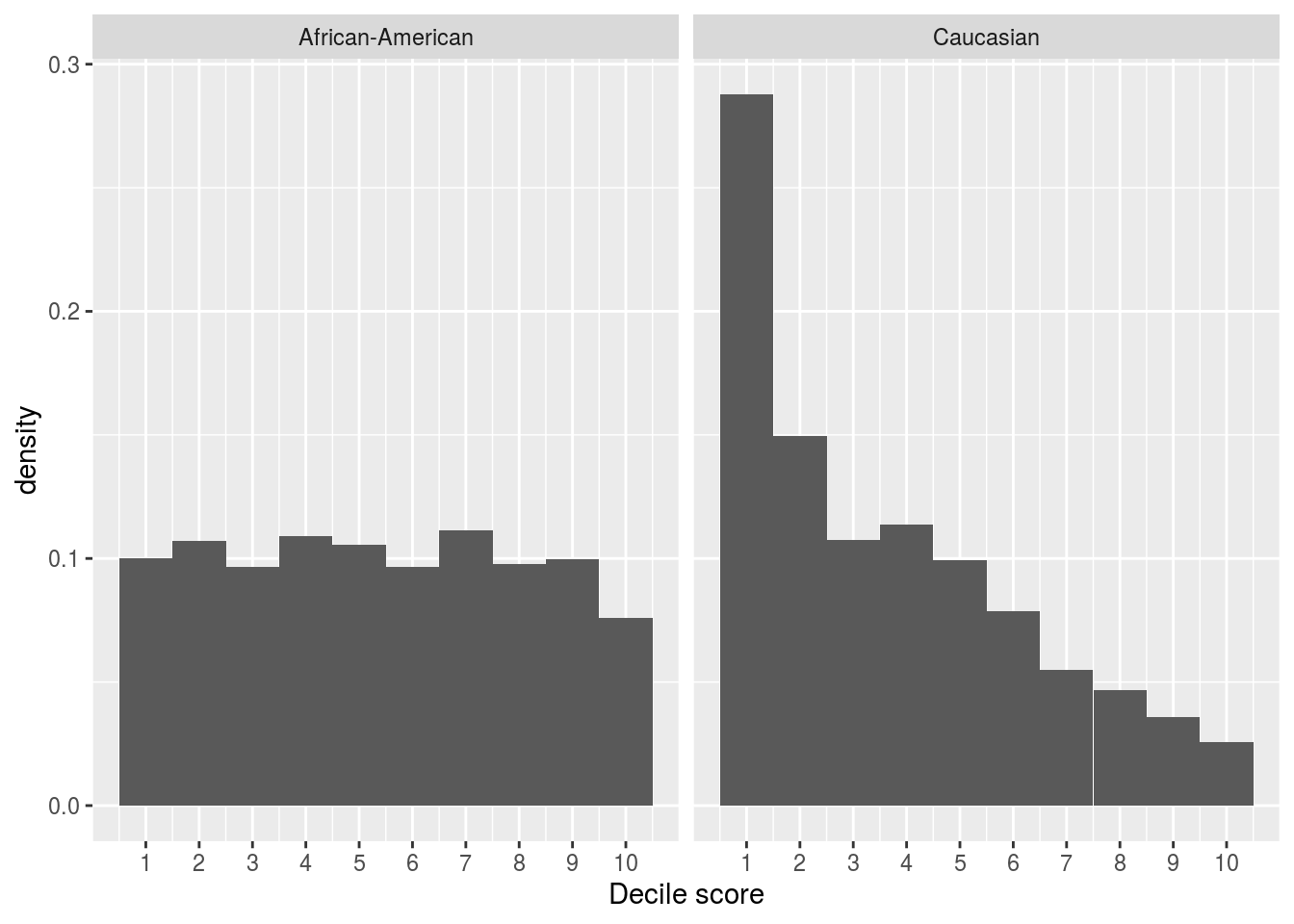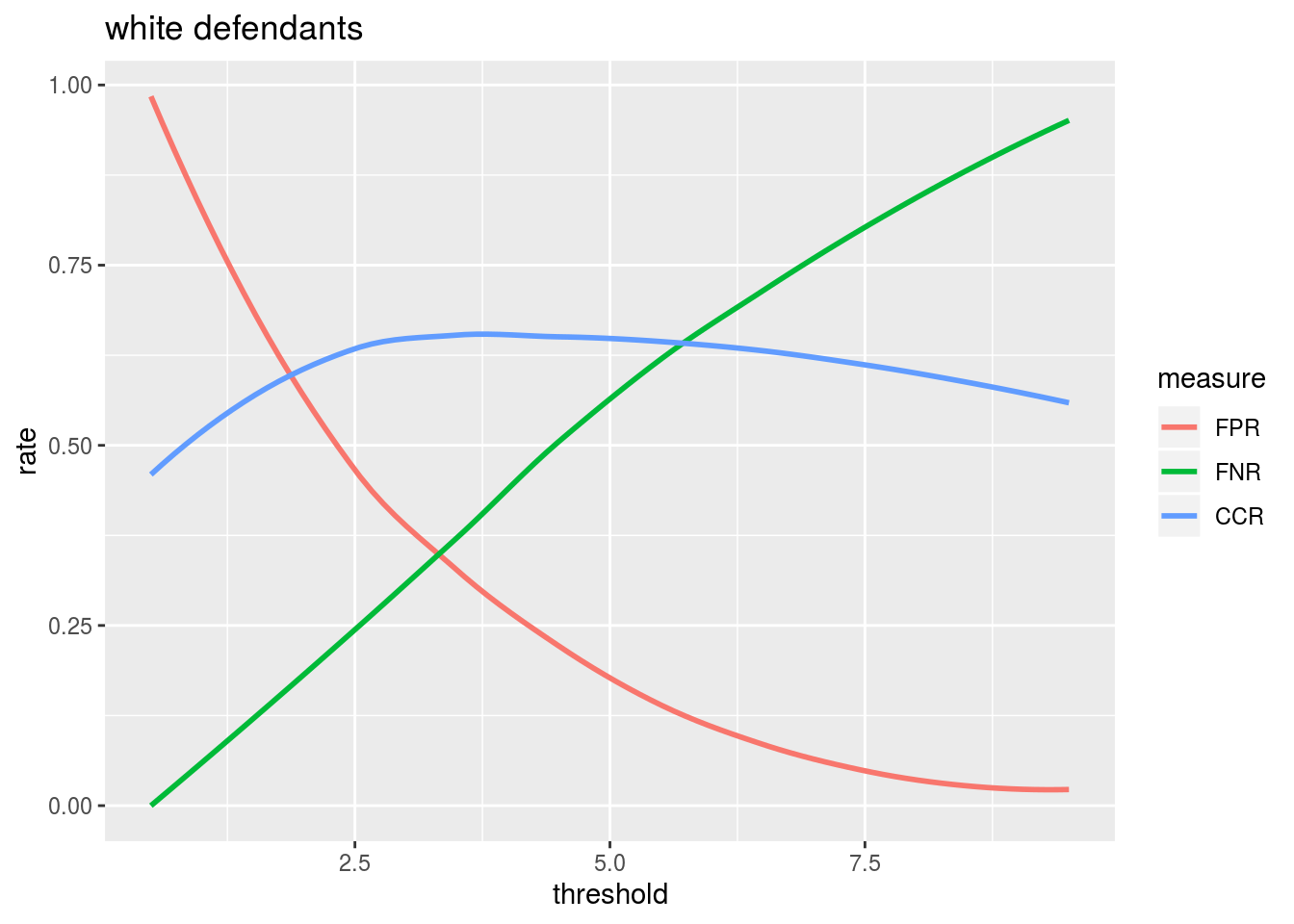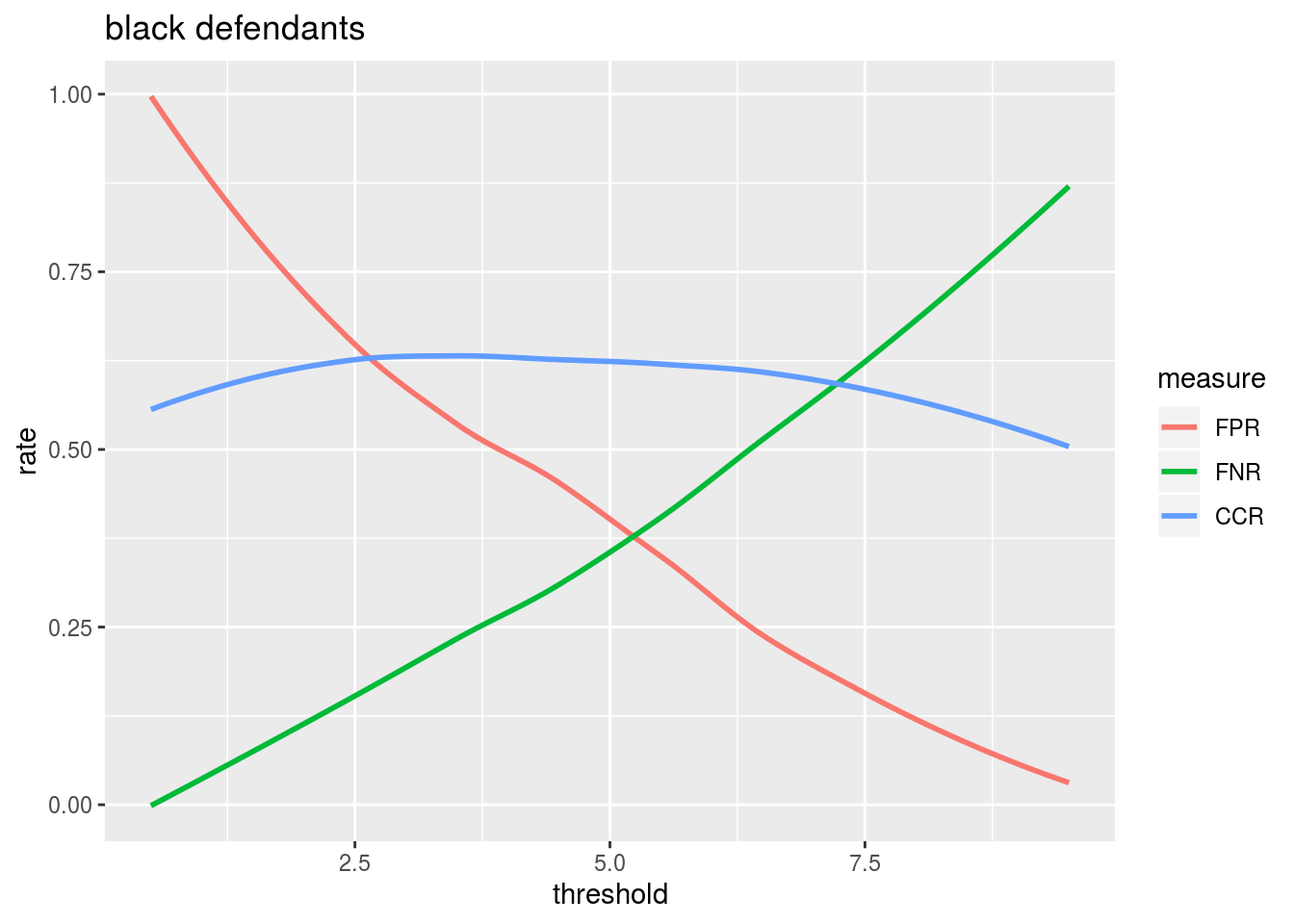We will be using the dataset at https://github.com/propublica/compas-analysis/raw/master/compas-scores-two-years.csv. Reading it in:

``````set.seed(0)

First, we’ll obtain the set we’ll be working with:

``````idx <- sample(1:nrow(compas))

idx.train <- idx[1:5000]
idx.test <- idx[5001:6000]
idx.valid <- idx[6001:7000]

compas.train <- compas[idx.train,]
compas.test <- compas[idx.test,]
compas.valid <- compas[idx.valid,]``````

### Part 1: Comparing the scores of black and white defendants

We first explore whether white and black defendants get the same COMPAS scores.

``````compas.bw <- compas.train %>% filter(race %in% c("African-American", "Caucasian"))

ggplot(compas.bw, mapping = aes(x = decile_score)) +
geom_histogram(bins = 10, mapping = aes(y = ..density..)) +
scale_x_continuous(breaks = c(1:10)) +
facet_wrap(~race)  +
xlab("Decile score")``````For African-American defendants, the distribution of the scores is approximately uniform. For Caucasian defendants, many more get low scores than high scores.

### Part 2: Initial evaluation of the COMPAS scores

Here, we are computing the FPR, FNR, and correct classification rate for different populations. First, we’ll define functions to compute the quantities needed.

``````get.FPR <- function(compas.set, thr){
sum((compas.set\$decile_score >= thr) & (compas.set\$is_recid == 0))/sum(compas.set\$is_recid == 0)
}

get.FNR <- function(compas.set, thr){
sum((compas.set\$decile_score < thr) & (compas.set\$is_recid == 1))/sum(compas.set\$is_recid == 1)
}

get.CCR <- function(compas.set, thr){
mean((compas.set\$decile_score >= thr) == compas.set\$is_recid)
}``````

Compute the sets we need:

``````compas.valid.b <- compas.valid %>% filter(race == "African-American")
compas.valid.w <- compas.valid %>% filter(race == "Caucasian")``````

Now, we can compute the numbers:

``````thr <- 5

fps <- c(get.FPR(compas.valid.b, 5), get.FPR(compas.valid.w, 5), get.FPR(compas.valid, 5))
fns <- c(get.FNR(compas.valid.b, 5), get.FNR(compas.valid.w, 5), get.FNR(compas.valid, 5))
ccr <- c(get.CCR(compas.valid.b, 5), get.CCR(compas.valid.w, 5), get.CCR(compas.valid, 5))

rates <- data.frame(FPR = fps, FNR = fns, CCR = ccr)
rownames(rates) = c("black", "white", "all")
rates``````
``````##             FPR       FNR       CCR
## black 0.4560669 0.3164983 0.6212687
## white 0.2254335 0.5070423 0.6476190
## all   0.3220676 0.4104628 0.6340000``````

We can see that the scores do not satisfy false positive parity and do not satisfy false negative parity. The scores do satisfy classification parity. Demographic parity is also not satisfied.

### Part 3: Altering the threshold

We will now see how changing the threshold influences the false positive, false negative, and correct classification rates.

``````get.rates <- function(thr, compas.set){
c(get.FPR(compas.set, thr), get.FNR(compas.set, thr), get.CCR(compas.set, thr))
}

thrs <- seq(0.5, 9.5, 1)
rates.w <- sapply(thrs, FUN=get.rates, compas.valid.w)
rates.b <- sapply(thrs, FUN=get.rates, compas.valid.b)
rates.all <- sapply(thrs, FUN=get.rates, compas.valid)

plot.rates <- function(thrs, rates, caption){
rates.df <- data.frame(threshold = thrs, FPR = rates[1,], FNR = rates[2,], CCR = rates[3,])
rates.df.tidy <- melt(rates.df, 1) %>% select(threshold = threshold, measure = variable, rate = value)
ggplot(rates.df.tidy, mapping = aes(x = threshold, y = rate, color = measure)) +
geom_smooth(se = F, method = "loess") + labs(title = caption)
}``````

We can now get three figures for the different demographics (let’s forego using facets again):

``plot.rates(thrs, rates.w, "white defendants")````plot.rates(thrs, rates.b, "black defendants")````plot.rates(thrs, rates.all, "all defendants")``